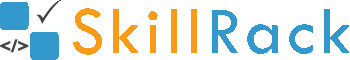ProgramID- 9233SkillRack
Reverse Even Integers

The program must accept N integers as the input. The program must print all the even integers among the N integers in reverse order as the output. If there is no even integer then the program must print -1 as the output.

Boundary Condition(s):
1 <= N <= 100
1 <= Each integer value <= 10^5

Example Input/Output 1:
Input:
5
1 2 5 7 8

Output:
8 2

Example Input/Output 2:
Input:
4
1 3 5 7

Output:
-1

Max Execution Time Limit: 1000 millisecs